Simulation of Structural Collapse with Coupled Finite Element-Discrete Element Method Xinzheng Lu1, Xuchuan Lin1 and Lieping Ye1 1Department of Civil Engineering, Tsinghua University, Beijing 100084, P.R. China Proc. Computational Structural Engineering, Yuan Y, Cui JZ and Mang H(eds.), Jun. 22-24, 2009, Springer, Shanghai:127-135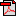Download PDF version Abstract. Structural progressive collapse is a great threat to life safety and therefore it is necessary to study its mechanism in detail. Numerical simulation is significant to study the whole process of progressive collapse in structural level. Since collapse is a complicated procedure from continuum into discrete fragments, numerical model should be competent in nonlinear deformation before collapse and breaking and crashing of fragments after collapse. Coupled Finite element-discrete element method on simulating structural progressive collapse is proposed to meet the requirements. Relatively accurate models, such as fiber model and multi-shell shell model, are introduced to construct the finite element model of structure. In the analysis, the failed finite elements will be removed and replaced with granular discrete elements according to the criteria of equivalent total mass and volume so that the impacting and heaping of fragments can be taken into account. The sample with the coupled method shows that this method not only possesses the advantages of finite element method but also simulates the behavior of fragments well. Keywords: Finite element, discrete element, coupling calculation, progressive collapse, numerical model.
 3.1 Generation of granular discrete element Once a four-node quad finite element is deactivated (removed from the model), nine granular discrete elements will be generated instead of this quad element, shown as figure 1. The generation process keeps the equivalent total mass and volume and the initial motion states of every discrete element, such as coordinates, velocities and accelerations are obtained with linear interpolation based on the four nodes of the deactivated finite element.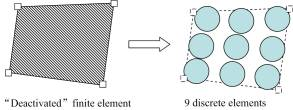Figure 1 The ��deactivated�� element is replaced with 9 discrete elements 3.2 Motion law of granular discrete element The sphere element can be regarded as a particle when moving, the motion equation at the moment ti is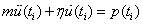(1) where m is mass of the discrete element, p is vector of external forces at ti, u is displacement vector at ti, �� is damping coefficient. Central difference method is applied to compute the motion state. If the time step is constant, that is ∆ti=∆t��we get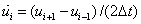(2)(3) By substitution of Eq. (2) and Eq. (3) into Eq. (1), we obtain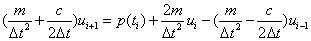(4) Then displacement, velocity and acceleration of a discrete element at every moment can be calculated through Eq.(2), Eq.(3) and Eq.(4). 3.3 Contact judgment Contact between two discrete elements happens if the distance between their spherical centers is no larger than the total length of their radiuses. The contact criterion (Wei et al 2008) for element i and element j is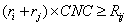(5)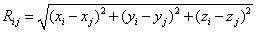(6) where ri��rj are radiuses of element i and element j respectively, Rij is the distance between the spherical centers of element i and element j , CNC is a coefficient relevant to the medium where elements soak, usually CNC��1.0 and CNC=1.0 in this paper. To simulate the impact of fragments to structures, contact between discrete element and finite element needs to be accomplished. The finite element is simplified as 9 spheres with the similar process in chapter 3.1 and thus, the contact between discrete element and finite element is transformed into that between two discrete elements. 4 Program implementation and example Coupled finite element-discrete element method was implemented through secondary development of commercial finite element software MSC.MARC 2005 and the details are as follows: (a) Deactivation of finite elements and generation of discrete elements. When a finite element is damaged, it will be deactivated (removed) by a user subroutine called UACTIVE. This process is controlled by the deactivating criteria (Lu et al 2008). Once the deformation of a finite element is too large, the element will be removed and the critical deformation can be determined in terms of maximum strain. The criteria defined in present work is the same as those in Lu et al 2008 and other criteria can also be defined by UACTIVE. The deactivated element is transformed into nine discrete elements as mentioned in chapter 3.1. The program gives the initial condition of discrete elements and record the motion state, mass and volume in the whole process. (b) Contact.

During collapse, impact and stack load of fragments have a significant effect on the stories below, so contact should be taken into account. There are three types of contact, contact between finite elements, contact between discrete elements and contact between finite elements and discrete elements. The first type of contact can be directly set in finite element software MSC.MARC and the second and third can be defined by user subroutine UBGITR. UBGITR will be called at the beginning of each iteration.

(c) Effect of impact and stack loads.

When the discrete elements collide with finite element structure, contact happens and user subroutine FORCDT will exert contact forces to the nodes of relevant finite elements.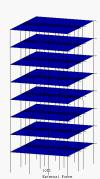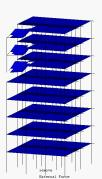(a) t=0.0s (b) t=1.0s (c) t=2.0s (d) t=3.0s

Figure 2 Collapse procedure simulated with finite element method (FEM)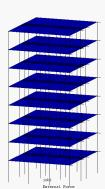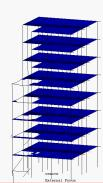(a) t=0.0s (b) t=1.0s (c) t=2.0s (d) t=3.0s

Figure 3 Collapse procedure simulated with coupled Finite element-discrete element method

A numerical example of an eight-story frame is carried out to observe the effect of coupled finite element-discrete element method. Columns and beams are modeled with fiber beam element model and slabs with multi-layer shell element model. Progressive collapse occurred after the corner column of the fifth story was removed. Two methods were applied to simulate collapse, one is FEM and the other is coupled finite element-discrete element method. The results from FEM are listed in figure 2 and those from coupled finite element-discrete element method (F/DEM) are listed in figure 3. It is noted that discrete elements can not be seen in Fig.11 because the program hasn��t support this function. At the beginning of collapse (before t=2.0s), structural response of FEM is similar to that of F/DEM. When t=1.0s, the slabs above the removed column break from the slabs around and drop off. When t=2.0s, the fallen slabs crash through the fifth floor. However, these two methods appear distinct results at 3.0s. With FEM, the progressive collapse is stopped after the fourth floor slabs drop. With F/DEM, all the floor slabs below the removed column are smashed. It is shown that the model based on F/DEM shows a better simulation on impact and stack load in the process of progressive collapse than that based on FEM.

5 Conclusions

Numerical simulation is an important tool to study progressive collapse. Collapse of structure is a complicated process from continuum into discrete bodies, which put forward requirements for the numerical model. The model should not only simulate the nonlinear behaviors before collapse, but also simulate impact and stack load of fragments during the collapse. Finite element method has advantages in simulating continuum while discrete element method in simulating the non-continuous behaviors after collapse starts. Coupled finite element-discrete element method (F/DEM) is proposed and implemented based on existing finite element software. Keeping the advantages of FEM, the method applies DEM to simulate the fragments in collapse. The numerical results show that this coupled FEM-DEM method has a better simulation on impact and stack load in progressive collapse than FEM.

Acknowledgements

This research is supported by National Science Foundation of China (No. 50808106 & 90815025)

References

Dong Qin, Li-chu Fan(2001). Numerical simulation on collapse process of reinforced concrete structures. Journal of Tongji University, 29(1), 80-83 [in Chinese].

Yu-zhu Cui, Chu-han Zhang,et al (2002). Numerical modeling of dam-foundation failure and simulation of arch dam collapse. Shuili Xuebao, 6,1-8 [in Chinese].

Li-ming Sun, Dong Qin, Li-chu Fan (2002). A new model for collapse analysis of reinforced concrete. China Civil Engineering Journal, 35(6), 53-58 [in Chinese].

D. Isobe, M. Tsuda(2003). Seismic collapse analysis of reinforced concrete framed structures using the finite element method. Earthquake Engineering and Structural Dynamics, 32, 2027-2046.

Gang Xuan, Xiang-lin Gu, Xi-lin L�� (2003). Numerical analysis of collapse process for RC frame structures subjected to strong earthquakes. Earthquake Engineering and Engineering Vibration, 23(6), 24-30 [in Chinese].

A. Munjiza, T. Bangash, N.W.M. John(2004). The combined finite-discrete element method for structural failure and collapse. Engineering Fracture Mechanics, 469-483.

Qiang Wang, Xi-lin L��(2004). Application of the DEM to the seismic response analysis of frame structures. Earthquake Engineering and Engineering Vibration, 24(5), 73-78 [in Chinese].

K. Khandelwal, and S. El-Tawil(2005). Multiscale computational simulation of progressive collapse of steel frames. Proceedings of the ASCE Structures Congress, May 2005.

Ji-jun Miao, Xiang-lin Gu, et al (2005). Numerical simulation analysis for the collapse response of masonry structures under earthquakes. China Civil Engineering Journal, 38(9), 45-52 [in Chinese].

Jing-bo Liu, Yang-bing Liu, Jian-guo Yang (2007). Simulation of earthquake ruins structure for the Earthquake Rescue Training Base of China. Journal of Disaster Prevention and Mitigation Engineering, 27(suppl.), 428-432 [In Chinese].

Yan-chao Shi, Zhong-xian Li, Hong He(2007). Numerical analysis of progressive collapse of reinforced concrete under blast loading. Journal of PLA University of Science and Technology, 18(6), 652-658 [in Chinese].

Xun-liu Wang, Xin-zheng Lu, Lie-ping Ye(2007). Numerical Simulation for the Hysteresis Behavior of RC Columns under Cyclic Loads. Engineering Mechanics, 24(12),76-81 [in Chinese] [in Chinese].

Feng-lei Zhao (2008). Simulation analysis for collapse of reinforced concrete frame structures under earthquake based on the discrete element method. Master thesis of Tongji University, Shanghai [in Chinese].

Long-hai Wei, Chun-guang Chen, et al(2008). Study on three-dimensional discrete element and parameter adoption. Journal of Chongqing Jiaotong University (Natural Science), 27(4), 618-621,629.

Xin-zheng Lu, Xu-chuan Lin,et al (2008). Numerical Simulation for the progressive collapse of concrete building due to earthquake. The 14th World Conference on Earthquake Engineering, Qctober 12-17, 2008, Beijing, China.